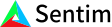1. Bob Anderson
×
IntuitiveML

## What is Artificial Intelligence? What is Machine Learning?

May 26, 2020

Artificial intelligence is intelligence that is demonstrated by machines, not people. In reality, the term is usually used to describe how computers mimic the different parts of human intelligence such as planning, learning, reasoning, and so on.

Machine learning on the other hand is the part of artificial intelligence solely focused on learning. What we mean by learning here is that instead of programming a specific way of doing a task, the machine is instead supposed to figure out how to do the task from examples.

What is machine learning used for? We use machine learning when we don’t know how to solve the problem programmatically – like when we are trying to figure out what is in an image. Think about it – if we are trying to identify if there is a cat in a picture, how would we go about doing that? There are tons of different cat species and breeds that can be of vastly different sizes, colors, and orientations. Additionally, the lighting of pictures can be different, the distance from the cat to the camera could be different, and a million other factors. How are we going to try to identify the infinite variations of cats from just the pixels of an image? If we were to try to solve this by programming, could we even list all of the different possible variations and things that we need to look out for? How long would it take to program by hand? What if we wanted to also identify the breed of the cat? How much more time would we need to solve that? With machine learning, instead of manually solving the problem, we can just feed a list of examples into our algorithm and expect our algorithm to figure out how to solve our problem. To some degree of accuracy, of course. For most machine learning tasks there are seemingly infinite variations of examples, and there is no way for a finite size dataset to cover all of the different examples. So there is always going to be some degree of accuracy for any given problem – whether that is 50% or 90% depends on how good the data is and how good the machine learning algorithm is.

How does machine learning work? Well, first off, for any given problem, we collect a whole lot of examples (like a million examples!) for that problem and then break each example up into two parts: the input and the output. We then assume that for any given input, there is some function that will give us the correct output.

We then go through all of the input-output pairs in our dataset of examples and for each pair, we modify the function so that the function is just a little bit better at predicting the output given the corresponding input. Why modify just a little bit? Why not just modify the function so that it just automatically predicts the output given the input? Well if we do that for every example, we will just forget the previous example and memorize the current one, which ultimately means that we aren’t learning anything. Instead, by slowly adjusting the function we can iteratively get closer and closer to the actual function as long as we have enough examples.

Of course, the quality of examples matters as well, because if a large number of examples are labeled incorrectly, then the algorithm will try to learn those incorrect examples, which will lower the performance. There are a couple of different ways of trying to handle the problem of data quality, but more on that in another video. :)